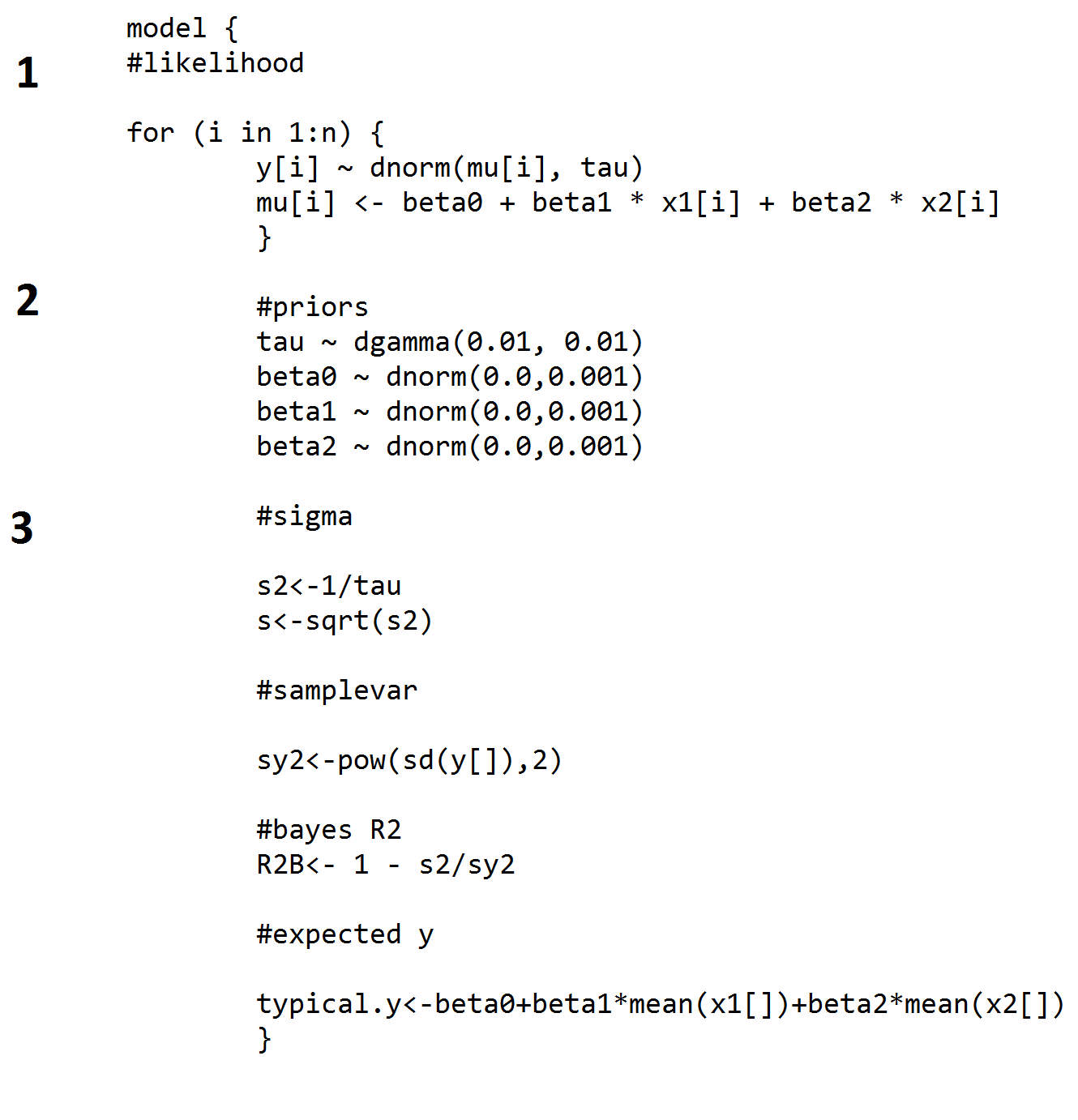# RJAGS: how to get started

## Solutions

Developed by Naomi Schalken, Lion Behrens and Rens van de Schoot

This tutorial expects:

• Basic knowledge of correlation and regression
• An installed version of R on your electronic device
• Basic knowledge of R

⇒ If you feel like you need a refresher of your R skills, have a look at R: how to get started

This tutorial provides the reader with a basic introduction to the software package RJags (Plummer, Stukalov & Denwood, 2016). RJags is a free package that can be used within the R environment. The reader will be guided through the process of downloading RJags and reading and running the R code to conduct a Bayesian regression analysis. Most of the written text consists of instructions. The exercises that are described in this document can also be found in the RegressionRjags.r file, which includes all the commands to run.

### Exercise 1

Open RStudio and open the .R file (RegressionRjags.r) that’s included in the folder. Then follow the next steps:

```install.packages("rjags")
install.packages("coda")
require(rjags)
require(coda)```

After defining the working directory where you have stored the regression.txt file, load the data into R's global environment.

```setwd("C:/your/folder/here")

Exercise 1c. Data Preparation

In order to run your model using RJags, the data set cannot be used as usual. In order to make it RJags-friendly, split the data in vectors for each variable and create a vector that holds the length of the data (N) by executing the code below.

```y <- data[,1]
x1 <- data[,2]
x2 <- data[,3]
n <- nrow(data)```

Exercise 1d. Define initial values for the model parameters of interest.

As you know, Bayesian inference consists of repeatedly sampling from a posterior distribution in order to get your parameter estimates and their variance. To get the sampling algorithm initialized in RJags, initial values for all model parameters (here tau, beta0, beta1 and beta2) need to be defined.

`model.inits <- list(tau=1, beta0=1, beta1=0, beta2=0)`

Exercise 1e. Iterations and Burn-in period

Additionally, you can define further aspects of the MCMC algorithm of repeated sampling. By specifying the number of iterations, you define how many samples should be taken from the posterior distribution overall. Since in first phases of the sampling process, sampled parameters are sometimes still quite distant from the posterior distributions maxima, a certain amount of iterations is often deleted and not taken into account for the evaluation of the results. Here, we define this Burn-in period to be half of the samples that are overall taken. Thus, our results will only depend on the second half of MCMC samples and will be more trustful than otherwise.

Furthermore, a possible but undesired scenario is that our result are overall dependent on the initial values that were the starting point for our MCMC iterations. To assess this, two independent chains of MCMC sampling can be requested, where the second chain uses different (and automatically created) initial values than specified. Of course, we want the results of both chains to resemble each other.

```iterations <- 1000
burnin <- floor(iterations/2)
chains <- 2```

### Exercise 2

In this exercise we continue with running the JAGS model. Before we run the model in R, open the Regression2JAGS.txt file in the folder. Take a look at the code in the file (see image below). The code is explained in three steps below.1. First, we specify the model, and start with the likelihood (a for-loop). Y is a normally distributed variable with mu (specified in the code) as the mean and tau as the precision. OpenBugs works with precision instead of variance. Precision is the inverse of the variance.

2. Underneath the likelihood we specify the priors to use for this model. The priors used here are relatively uninformative, with normal distributions for the intercept (beta0) and regression coefficients (beta1 and beta2), and a gamma distribution for the precision.

3. Then we define a few more parameters. First, the residual variance of y (s2), and the square root of this variance, the standard deviation (s). Below that we use a formula to retrieve the total variance of y (sy2) and use that to compute a “Bayesian R2”. Finally, we compute a typical y value based on the regression coefficients, using mean values for the observed x1 and x2.

Exercise 3a. Run the JAGS model. The txt. file holds the Bugs model input (excluding data and inits parts). Data is a list of the vectors you specified in Exercise 1. N.chains are the number of chains you specified in Exercise 1.

```model.fit <- jags.model(file="Regression2JAGS.txt",
data=list(n=n, y=y, x1=x1, x2=x2),
inits=model.inits, n.chains = chains)```

Exercise 3b. Run the model for a certain number of MCMC iterations and extract random samples from the posterior distribution of parameters of interest. First specify the model object and then c() the parameters you would like to have information on. N.iter is the number of iterations to monitor. We specified this number in Exercise 1.

``` model.samples <- coda.samples(model.fit, c("beta0", "beta1", "beta2",
"R2B", "s2"), n.iter=iterations)```

Exercise 3c. Obtain summary estimates of the parameters.

` summary(window(model.samples, start = burnin))`

Exercise 3d. Obtain plots of the posterior distributions of the parameters.

plot(model.samples, trace=FALSE, density = TRUE)

Question: Do the posterior distributions look like you expected them?

### References

Plummer, M., Stukalov, A., & Denwood, M. (2016). Bayesian Graphical Models using MCMC.

### Other software exercises you might be interested in

MPLUS

How to get started

First Bayesian Inference

How to avoid and when to worry about the misuse of Bayesian Statistics

------------------------------------------

SPSS

How to get started

------------------------------------------

Lavaan

How to get started

------------------------------------------

Blavaan

How to get started

First Bayesian Inference

How to avoid and when to worry about the misuse of Bayesian Statistics

------------------------------------------

RJAGS

First Bayesian Inference

How to avoid and when to worry about the misuse of Bayesian Statistics

------------------------------------------

JASP

How to get started